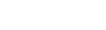MyDACOccurs when it is needed to put column values.

### Syntax

property OnGetColumnData: TGetColumnDataEvent;

### Remarks

Another way to load data is using the OnPutData event.

### Example

```procedure TfmMain.GetColumnData(Sender: TObject;
Column: TDAColumn; Row: Integer; var Value: Variant;
var EOF: Boolean);
begin
if Row <= 1000 then begin
case Column.Index of
0: Value := Row;
1: Value := Random(100);
2: Value := Random*100;
3: Value := 'abc01234567890123456789';
4: Value := Date;
else
Value := Null;
end;
end
else
EOF := True;
end; ```